Courses

# Test: Solutions 2 - From Past 28 Years Questions

## 30 Questions MCQ Test Chemistry Class 12 | Test: Solutions 2 - From Past 28 Years Questions

Description
This mock test of Test: Solutions 2 - From Past 28 Years Questions for Class 12 helps you for every Class 12 entrance exam. This contains 30 Multiple Choice Questions for Class 12 Test: Solutions 2 - From Past 28 Years Questions (mcq) to study with solutions a complete question bank. The solved questions answers in this Test: Solutions 2 - From Past 28 Years Questions quiz give you a good mix of easy questions and tough questions. Class 12 students definitely take this Test: Solutions 2 - From Past 28 Years Questions exercise for a better result in the exam. You can find other Test: Solutions 2 - From Past 28 Years Questions extra questions, long questions & short questions for Class 12 on EduRev as well by searching above.
QUESTION: 1

### An ideal solution is formed when its components 

Solution:

For ideal solution,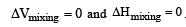QUESTION: 2

### All form ideal solution except 

Solution:

C2H5I and C2H5OH form non-ideal solution.

QUESTION: 3

### The relative lowering of the vapour pressure is equal to the ratio between the number of 

Solution:

According to Raoult's law, the relative lowering in vapour pressure of a dilute solution is equal to the mole fraction of the solute present in the solution.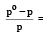mole fraction of solute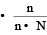QUESTION: 4

Which of the following aqueous solution has minimum freezing point ? 

Solution:

ΔTf = i × Kf × m Van't Hoff factor, i = 2 for NaCl, hence ΔTf = 0.02 Kf which is maximum in the present case.
Hence ΔTf is maximum or freezing point is minimum.

QUESTION: 5

Blood cells retain their normal shape in solution which are 

Solution:

Blood cells neither swell n or shrink in isotonic solution. As isotonic solutions have equal concentration therefore there is no flow of solvent is found and hence solvent neither enters nor flow out of the blood cells.

QUESTION: 6

Which of the following modes of expressing concentration is independent of temperature ? [1992,1995]

Solution:

The molality involves weights of the solute and the solvent. Since weight does not change with the temperature, therefore molality does not depend upon the temperature.

QUESTION: 7

Which one is a colligative property ? 

Solution:

Osmotic pressure is a colligative property.

QUESTION: 8

If 0.1 M solution of glucose and 0.1 M solution of urea are placed on two sides of the semipermeable membrane to equal heights, then it will be correct to say that 

Solution:

As both the solutions are isotonic hence there is no net movement of the solvent through the semipermeable membrane between two solutions.

QUESTION: 9

At 25°C, the highest osmotic pressure is exhibited by 0.1 M solution of 

Solution:

Conc. of particles in CaCl2 sol. will be max. as i = 3 is max.
Note : Glucose and Urea do not dissociate into ions, as they are nonelectrolytes.

QUESTION: 10

Which one of the following salts will have the same value of van’t Hoff factor (i) as that of K4[Fe (CN)6]. 

Solution:

K4 [Fe(CN)6] and Al2(SO4)3 both dissociates to give 5 ions or i = 5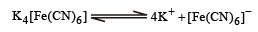and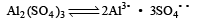QUESTION: 11

The number of moles of oxygen in one litre of air containing 21% oxygen by volume, in standard conditions, is 

Solution:

Percentage volume of oxygen = 21%. (Given)
∵ 100 ml of air contains = 21ml of O2
∴ Volume of oxygen in one litre of air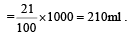Therefore no. of moles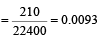(∵ volume of 1 litre of gas at S.T.P. is 22400 ml)

QUESTION: 12

Vapour pressure of benzene at 30°C is 121.8 mm.When 15 g of a non volatile solute is dissolved in 250 g of benzene its vapour pressure decreased to 120.2 mm. The molecular weight of the solute (Mo. wt. of solvent = 78) 

Solution:

Give vapour pressure of  pur e solute (P0) = 121.8 mm;
Weight of solute (w) = 15 g
Weight of solvent (W) = 250 g;
Vapour pressure of pure solvent (P) = 120.2 mm and Molecular weight of solvent (M) = 78 From Raoult’s law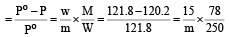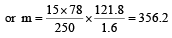QUESTION: 13

According to Raoult's law, relative lowering of vapour pressure for a solution is equal to  [1995

Solution:

Relative lowering of vapour pressure depends upon the mole fraction of solute.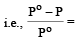mole fraction of solute

QUESTION: 14

The vapour pressure at a given temperature of an ideal solution containing 0.2 mol of a nonvolatile solute and 0.8 mol of solvent is 60 mm of Hg. The vapour pressure of the pure solvent at the same temperature is 

Solution:

According to Raoult's law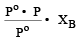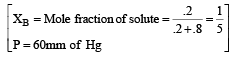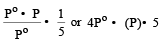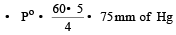QUESTION: 15

Which of the following 0.10 m aqueous solutions will have the lowest freezing point ? 

Solution:

Depression in F.P. ∝ No. of particles.
Al2(SO4)3 provides five ions on ionisation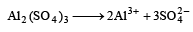while KCl provides two ions
KCl     K    Cl
C6H12O6 and C12H22O11 are not ionised so they have single particle.
Hence, Al2(SO4)3 have maximum value of depression in F.P or lowest F.P

QUESTION: 16

A 5% solution of cane sugar (mol. wt. =342) is isotonic with 1% solution of a substance X. The molecular weight of X is 

Solution:

Osmotic pressure of 5% cane sugar solution 5% cane sugar solution means 100ml of solution contain cane sugar = 5g

∴    1000 of solution contain cane sugar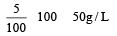Similarily 1% is solution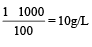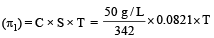Osmotic pressure of 1% solution of susbtance  (π2)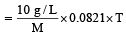As both are isotonic, So
π1 = π2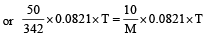∴  M (mol. wt. of X)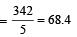QUESTION: 17

The vapour pressure of a solvent decreased by 10mm of mercury when a non-volatile solute was added to the solvent. The mole fraction of the solute in the solution is 0.2. What should be the mole fraction of the solvent if the decrease in the vapour pressure is to be 20mm of mercury?

Solution:

According to Raoult's law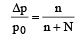(mole fraction of solute)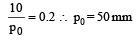For other solution of same solvent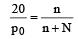(Mole fraction of solute)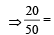mole fraction of solute

⇒   Mole fraction of solute = 0.4 As mole praction of solute + mole traction of solvent = 1

Hence, mole fraction of solvent = 1 – 0.4    = 0.6

QUESTION: 18

Which of the following statements, regarding the mole fraction (x) of a component in solution, is incorrect? 

Solution:

Mole fraction of any component A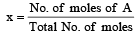As total no. of moles > no. of moles of A thus x can never be equal to one on zero.

QUESTION: 19

Which of the following colligative property can provide molar mass of proteins (or polymers or colloids) with greatest precision ? 

Solution:

Molecular masses of polymers are best determined by osmotic pressure method .
Firstly because other colligative properties give so low values that they cannot be measured accurately and secondly, osmotic pressure measurements can be made at room temperature and do not require heating which may change the nature of the polymer.

QUESTION: 20

The beans are cooked earlier in pressure cooker, because 

Solution:

The beans are cooked earlier in pressure cooker because boiling point increases with increasing pressure.

QUESTION: 21

Molarity of liquid HCl will be, if density of solution is 1.17 gm/cc 

Solution:

Density = 1.17 gm/cc (Given)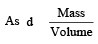volume = 1cc          ∴ mass = d = 1.17g

now molarity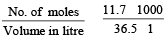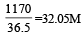QUESTION: 22

1 M, 2.5 litre NaOH solution is mixed with another 0.5 M, 3 litre NaOH solution. Then find out the molarity of resultant solution 

Solution:

From molarity equation M1V1 + M2V2 = MV
1× 2.5 + 0.5 × 3 = M × 5.5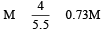QUESTION: 23

A solution con tains non -volatile solute of molecular mass M2. Which of the following can be used to calculate the molecular mass of solute in terms of osmotic pressure ? 

Solution: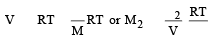QUESTION: 24

A solution containing components A and B follows Raoult's law when 

Solution:

These two components A and B follows the condition of Raoult’s law if the force of attraction between A and B is equal to the force of attraction between A and A or B and B.

QUESTION: 25

Formation of a solution from two components can be considered as 
(i) Pure solvent → separated solvent                              molecules, ΔH1
(ii) Pure solute   → separated solute                               molecules, ΔH2
(iii) Separted solvent & solute molecules → Solution,  ΔH3
Solution so formed will be ideal if

(a) ΔHsoln = ΔH1 + ΔH2 - ΔH3

(b) ΔHsoln = ΔH1 + ΔH2 + ΔH3

(c) ΔHsoln = ΔH1 - ΔH2 - ΔH​​​​​​​3

(d) ΔHsoln = ΔH3 - ΔH1 - ΔH​​​​​​​2

Solution:

For an ideal solution, ΔHmixing = 0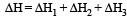(Accroding to Hess's law) i.e., for ideal solutions there is no change in magnitude of the attractive forces in the two components present.

QUESTION: 26

Camphoris often used in molecular mass determination because 

Solution:

Solvent having high cryoscopic constant can be used in determination of molecular mass by cryoscopic method.

QUESTION: 27

The vapour pressure of two liquids ‘P’ an d ‘Q’ are 80 and 60 torr, respectively. The total vapour pressure of solution obtained by mixing 3 mole of P and 2 mole of Q would be 

Solution:

Given V.PP = 80 torr  V.PQ = 60 torr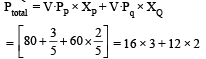Ptotal = 48 + 24 = 72 torr

QUESTION: 28

A solution of urea (m ol. mass 56 g mol-1) boils at 100.18°C at the atmospheric pressure. If Kf and Kb for water are 1.86 and 0.512 K kg mol-1 respectively, the above solution will freeze at 

Solution:

As ΔTf = Kf. m
ΔTb = Kb. m

Hence, we have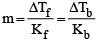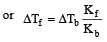= [ΔTb = 100.18 - 100 = 0.18°C]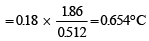As the Freezing Point of pure water is 0°C,
ΔTf = 0 –Tf
0.654 = 0 – Tf
∴  Tf = – 0.654 thus the freezing point of solution will be – 0.654°C.

QUESTION: 29

The mole fraction of the solute in one molal aqueous solution is: [2 00 5]

Solution:

One molal solution means one mole solute present in 1 kg (1000 g) solvent i.e., mole of solute = 1

Mole of solvent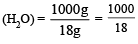Mole fraction of solute  =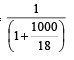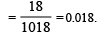QUESTION: 30

A solution of acetone in ethanol 

Solution:

A solution of acetone in ethanol shows positive deviation from Raoult's law. It is because ethanol molecules are strongly hydrogen bonded. When acetone is added, these molecules break the hydrogen bonds and ethanol becomes more volatile. Therefore its vapour pressure is increased.